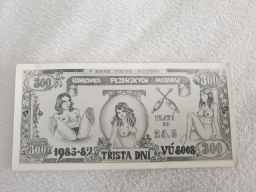# Remaining 36343

Nina is going skiing. She bought trousers with a quarter of the saved money, spent a third of the amount on a backpack, and a friend sold her skis for the remaining €100. How much € did Nina save?

x =  240

### Step-by-step explanation:

x/4+x/3+100=x

5x = 1200

x = 1200/5 = 240

x = 240

Our simple equation calculator calculates it.Did you find an error or inaccuracy? Feel free to write us. Thank you!

Tips for related online calculators
Need help calculating sum, simplifying, or multiplying fractions? Try our fraction calculator.
Do you have a linear equation or system of equations and looking for its solution? Or do you have a quadratic equation?